# In this section of the page I am going to talk about my R project

My project is about pollutant in Barcelon(Palaureial)

Te objectives of the project are:

The steps that I have followed to carry out this project are:

• Open Rstudio
• I followed the steps of tecnologies.net
• Something to keep in mind is that to make the graphs you need that the date/date column with time has to be called: "date", the class of the date has to be: "date" and the date with hours: "POSIXct".
• Unfortunedly I can't use the function spread() because the version of R that I used can't use the function spread() after using the function gather().
•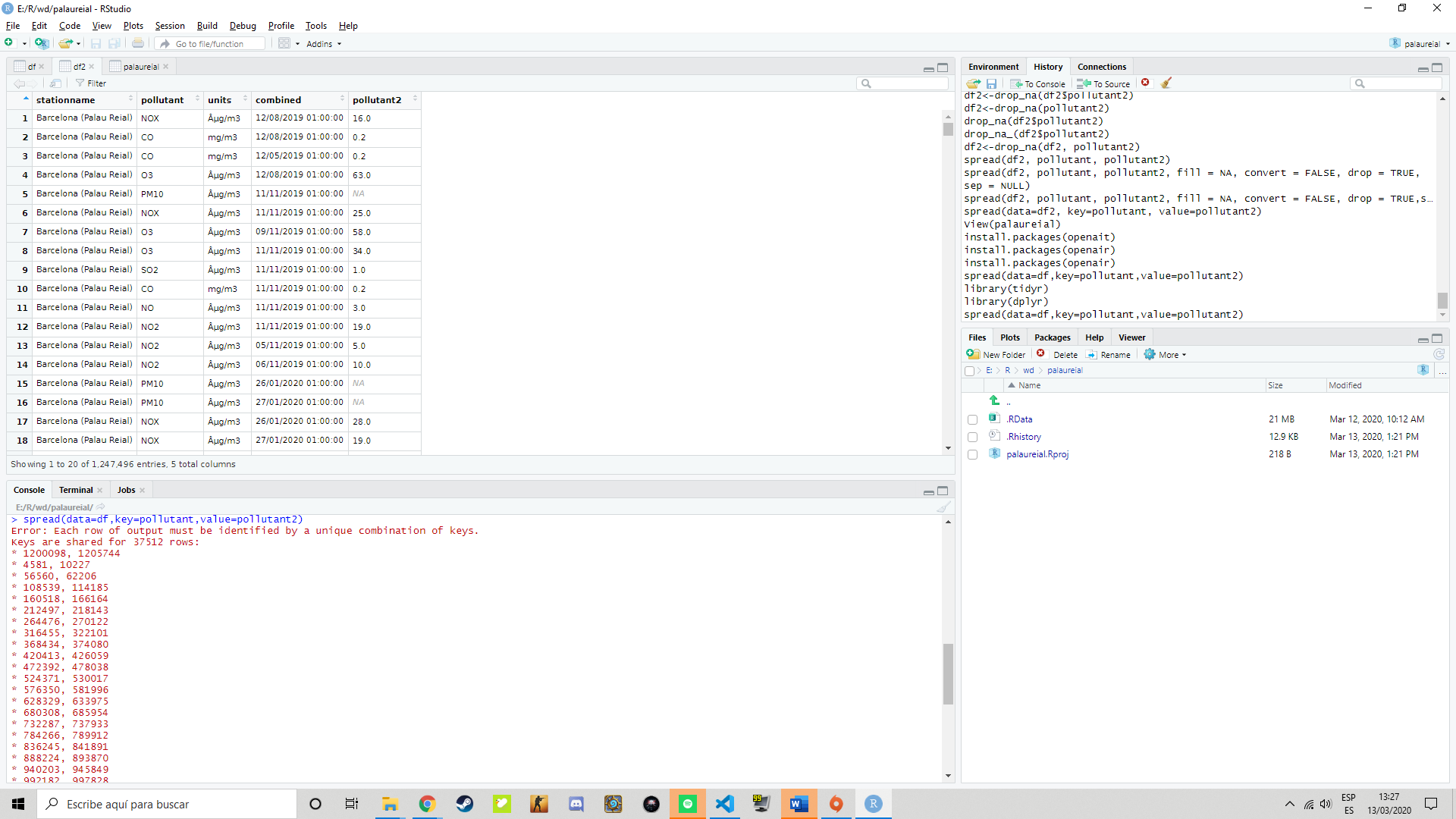• Then, I looked for a function similar to spread (), and found the pivot_wider() function.and this was the result.
•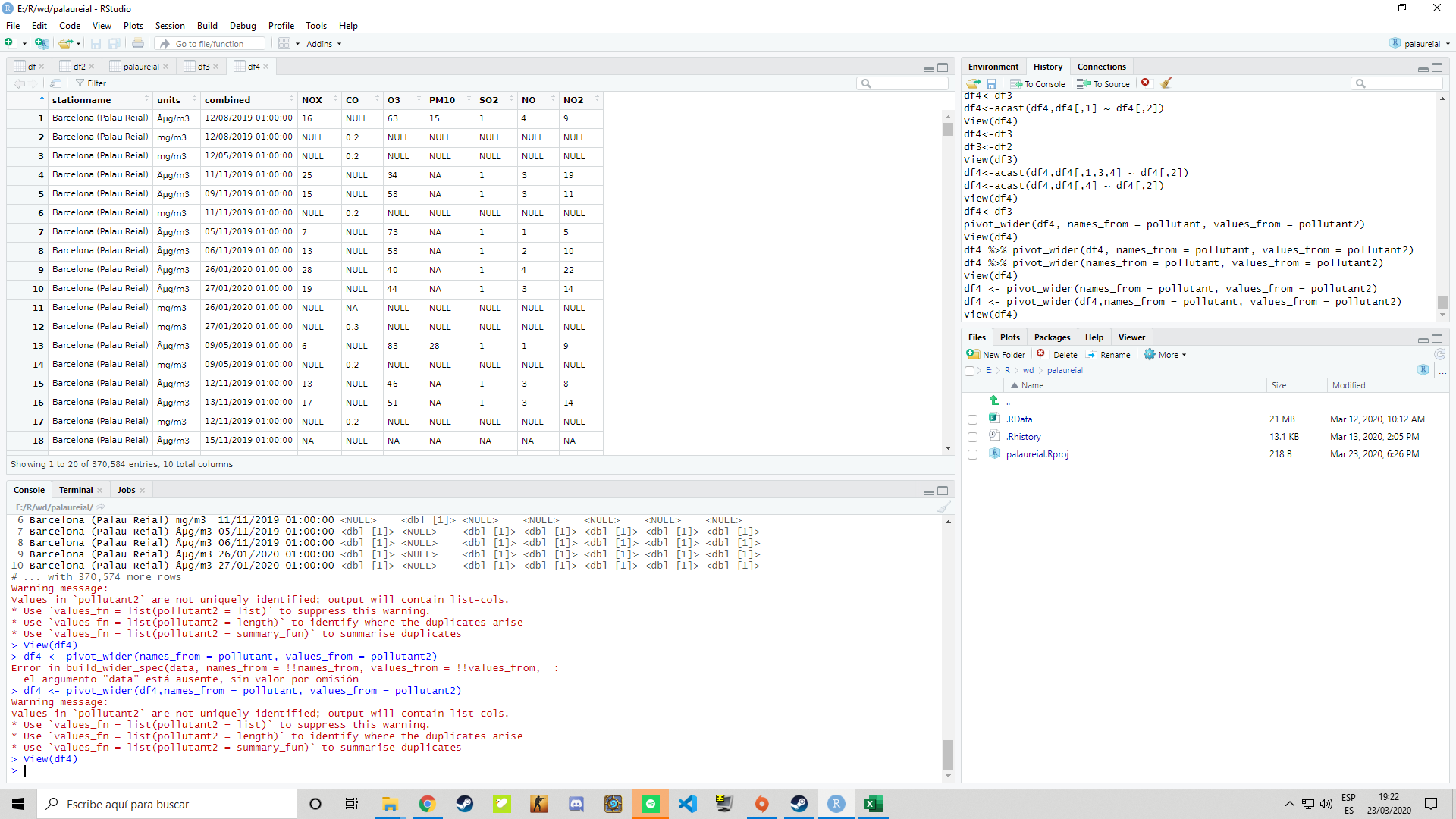• When I used the pivot_wider() function I ran into the problem that you I couldn't use a write.csv () because Rstudio recognizes pollutants as lists.
• To solve that provlem I do this:
•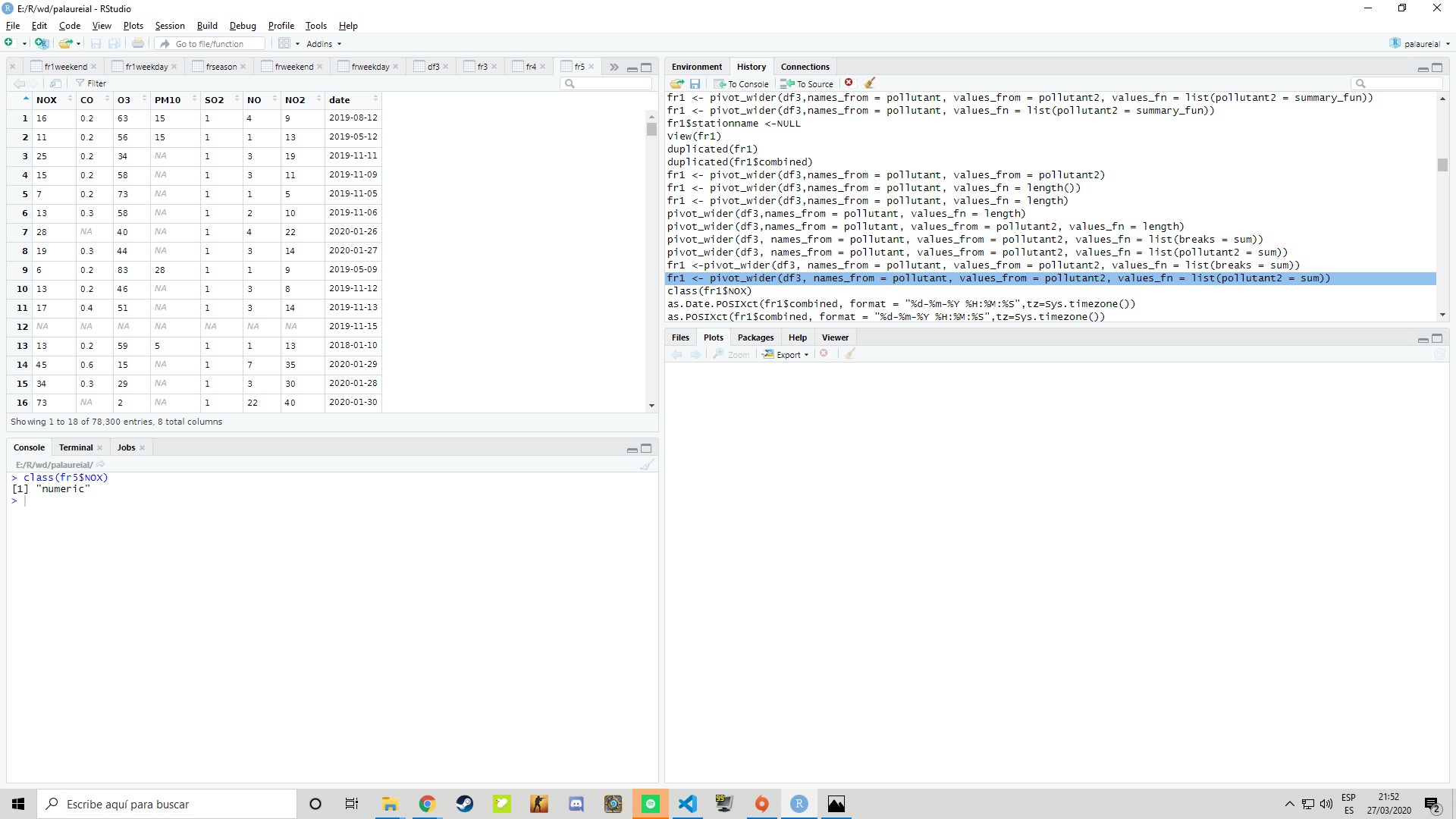• Once this is done, you must change the class of "combined" which is the date, for this I used the library lubridate.
• Finally we can start creating the graphics with openair.
• To create the graphics I followed the tecnologies.net instructions.
• For the first one I used the function "calendarPlot (fr2, pollutant = "NOX", year = "2012")" and this is the result:
•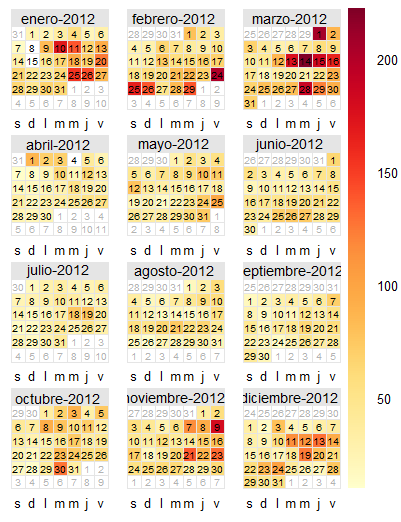• I decided to comparate the last one with the same type of graphic but with the data of 2019 using the function "calendarPlot (fr2, pollutant = "NOX", year = "2019")" and this is the result:
•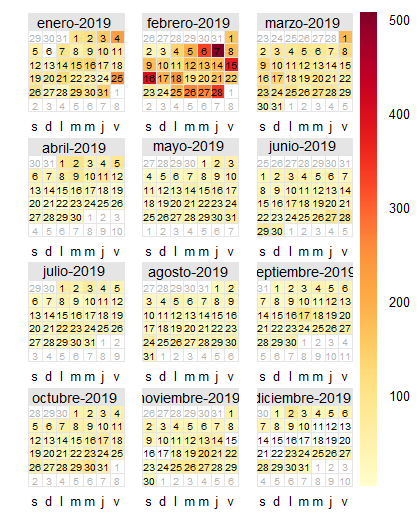• We can observe that in 2012 the limit was in 200 ug/m3 but in 2019 the limit was in 500 ug/m3, as a conclusion we can say that the NOx has increased a lot.
• For the next I used the function"timePlot(fr5, pollutant=c("NOX","O3","SO2","NO","NO2"))" to see a time plot of NOx, O3, SO2, NO and NO2.
•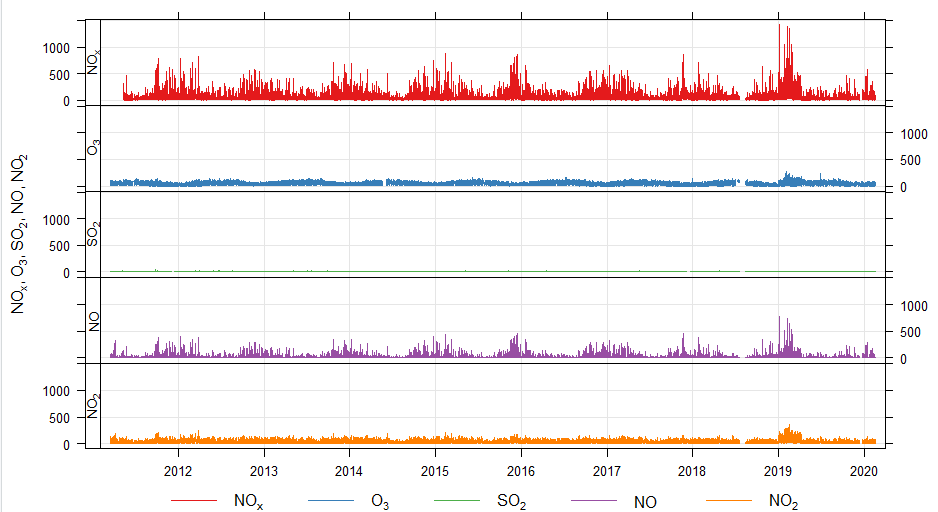• The next instrucion is to see compare a normalised level of NOx, NO2 and O3.
• "timePlot(fr3, pollutant = c("NOX”, “NO2”, "O3"),

+avg.time = “month”, normalise = “01/01/2019”, lwd = 4, lty = 1,

+group = TRUE, ylim = c(0, 300))".
•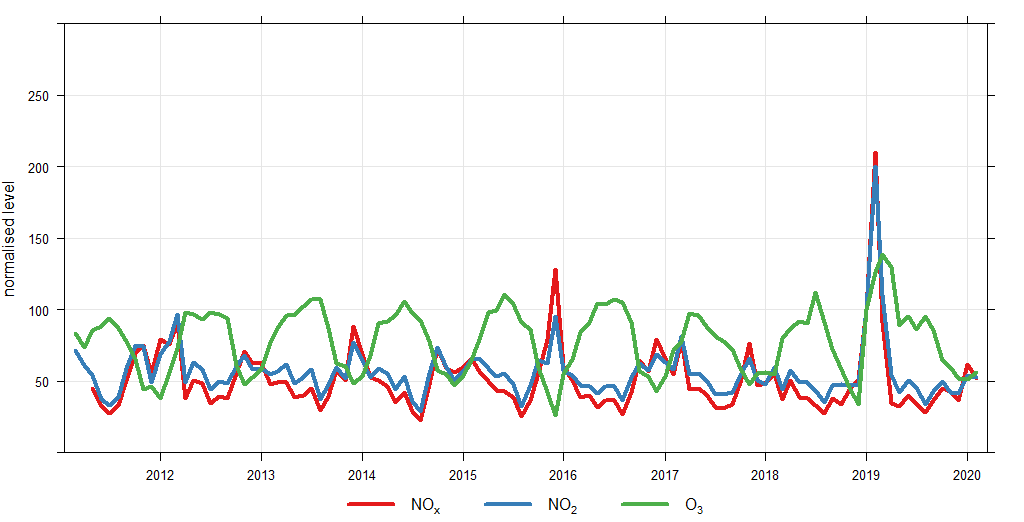• The next is a graphic about 2019 month plot of Palau reial NO2.
• The instruction is: "monthplot(fr5\$NO2, main=expression(paste("2019 Month plot of Palau Reial NO")), ylab="Conc (ug/m3)")".
•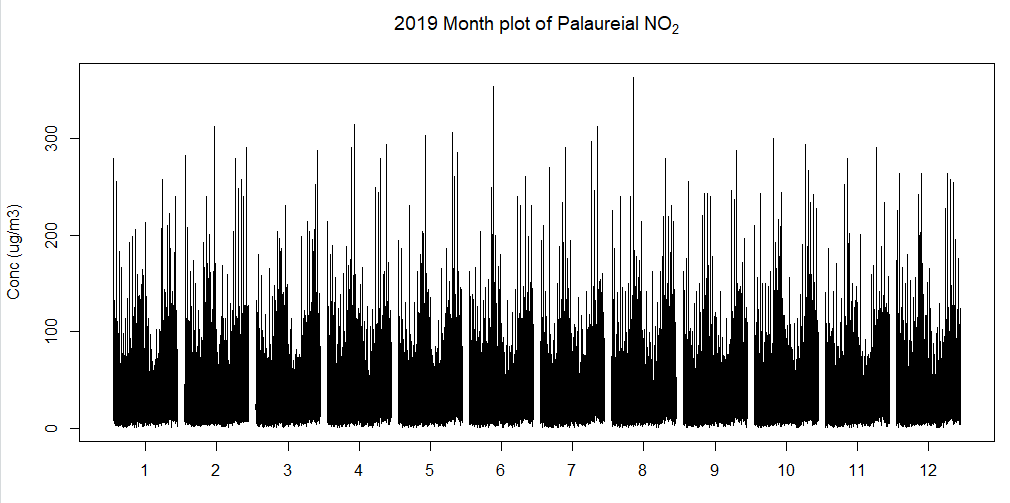• For the next I used the function "summaryPlot(fr4)" to have a summary of fr4 (my dataframe).
•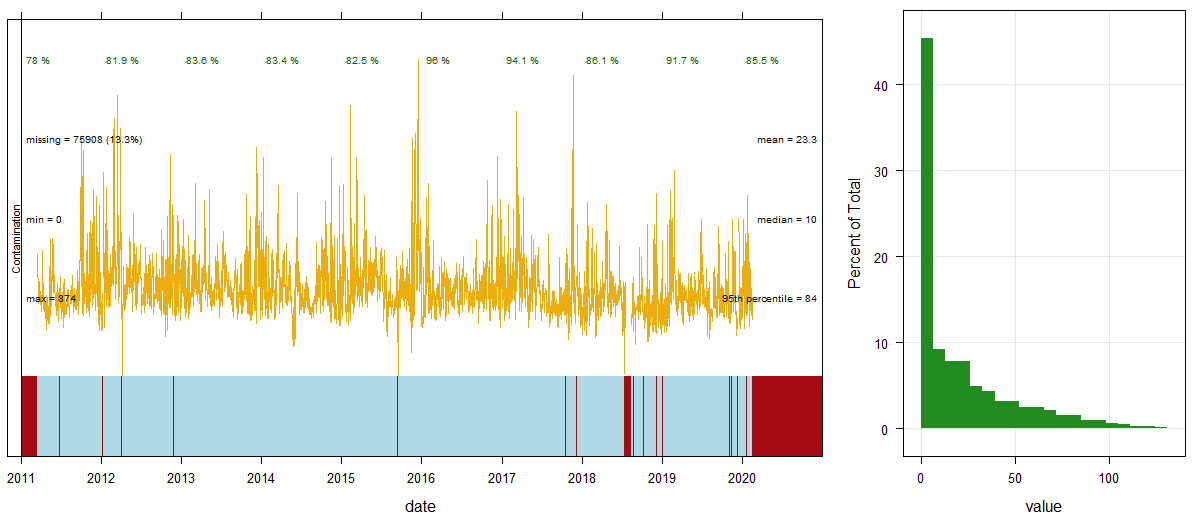• To see a trend level evolution of CO in Palau reial you have to use: "trendLevel(fr5, pollutant = "CO")".
•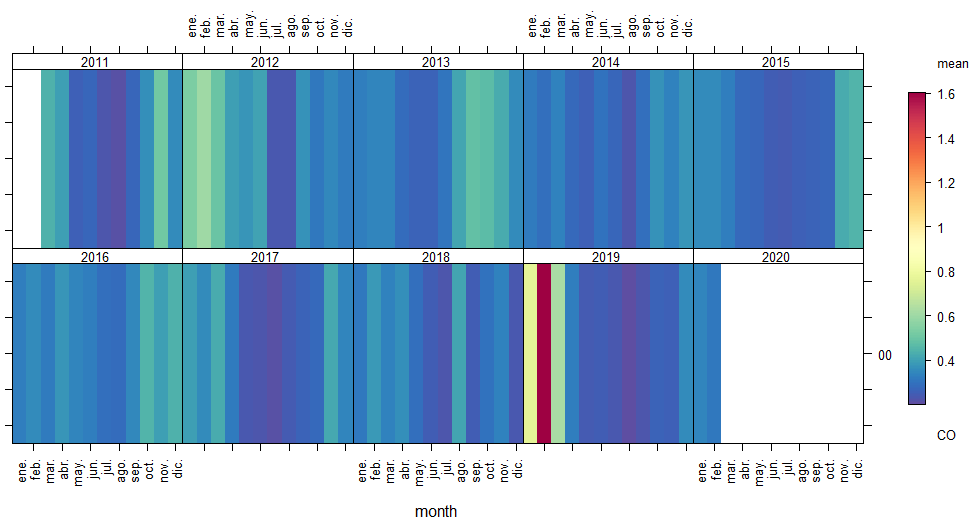• If you want to be more especific you need to use "trendLevel(fr5, pollutant = "NO2", x="hour",y="weekday",type="season",rotate.axis = c(0,45), cols = "heat",xlab="Hour of the day", ylab="Week day", main="NO2 evolution in seasons (Palau Reial)")".
•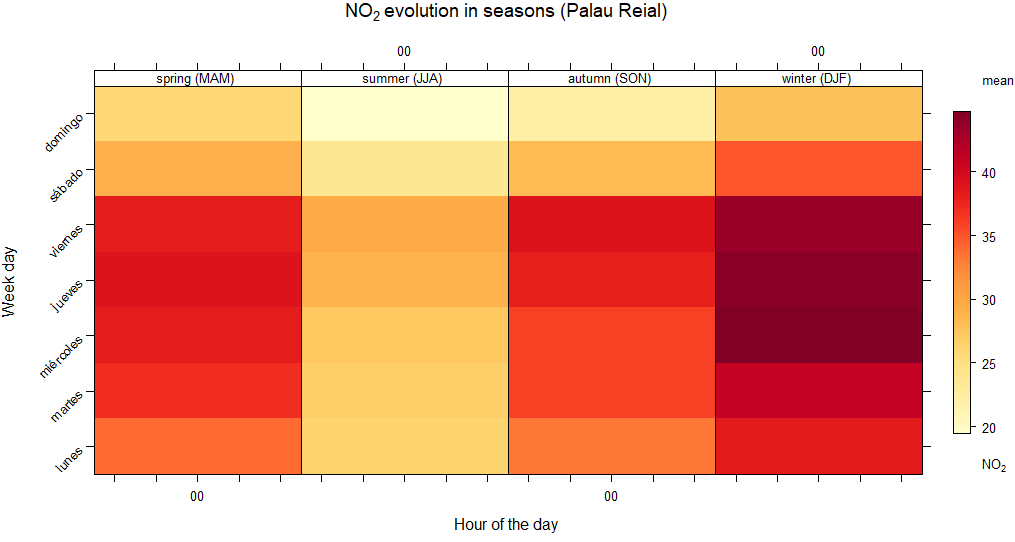• To see a graphic about the pollution in a especific year you have to use: timeVariation(fr2019, pollutant=c("NOX","CO","O3","PM10","SO2","NO","NO2"), normalise = TRUE)
•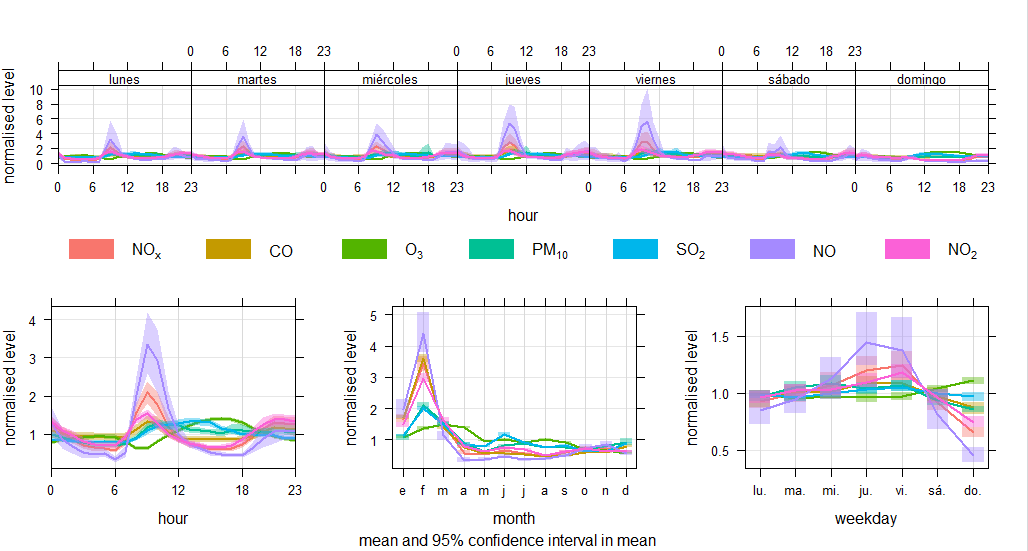• And finally to see a smooth trend of SO2 by percentiles you have to use: "smoothTrend(fr5,pollutant = "SO2",deseason = TRUE, statistic = "percentile", percentile = c(25,50,75,95))".
•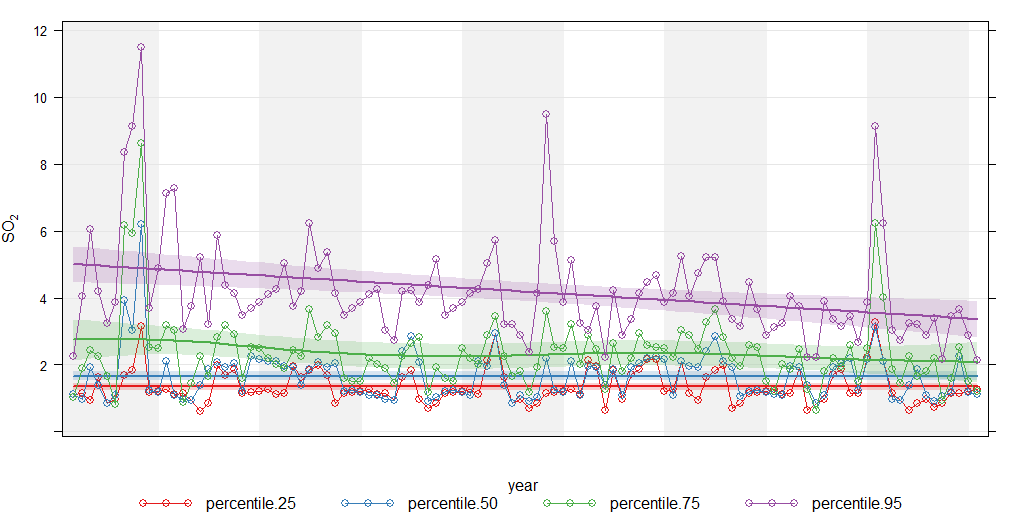As we can see, in Palau reial the limits established by the European Union are exceeded. Which should make us think about the future of the planet.

I tried to do the next part of the job but I didn't quite understand it I hope we can finish it all together in class.